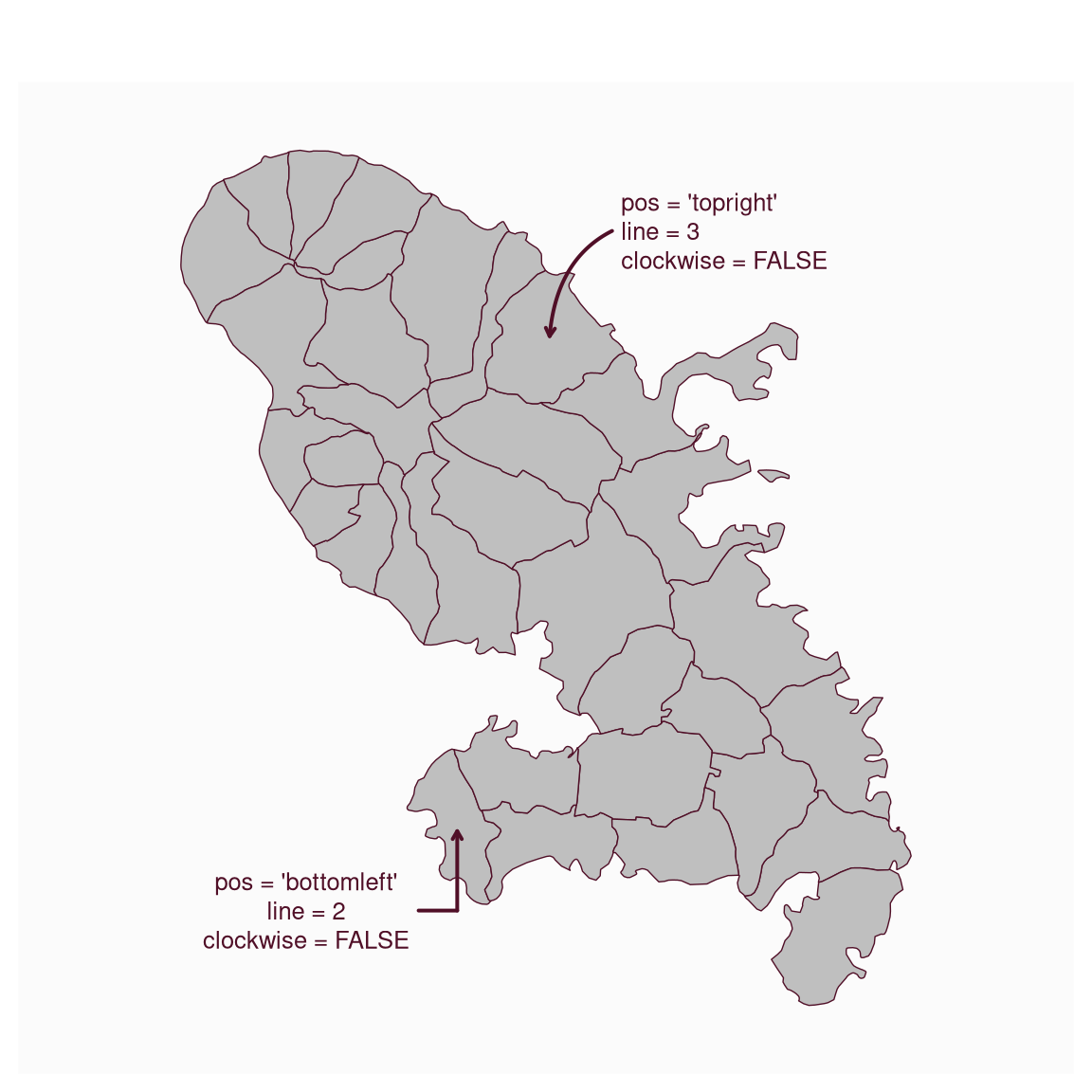Plot an annotation on a map.

mf_annotation(
x,
txt,
pos = "topright",
cex = 0.8,
col_arrow,
col_txt,
halo = FALSE,
bg,
s = 1,
...
)

## Arguments

x

an sf object with 1 row, a couple of coordinates (c(x, y)) or "interactive"

txt

the text to display

pos

position of the text, one of "topleft", "topright", "bottomright", "bottomleft"

cex

size of the text

col_arrow

arrow color

col_txt

text color

halo

add a halo around the text

bg

halo color

s

arrow size (min=1)

...

further text arguments.

## Value

No return value, an annotation is displayed.

## Examples

mtq <- mf_get_mtq()
mf_map(mtq)
mf_annotation(
x = c(711167.8, 1614764),
txt = "Look!\nImportant feature\nhere!",
pos = "bottomleft", cex = 1.2, font = 2,
halo = TRUE, s = 1.5
)

mf_annotation(
x = mtq[20, ],
txt = "This is less\nimportant",
cex = .7, font = 3, s = 1.3
)